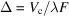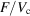International
Tables for
Crystallography
Volume B
Reciprocal space
Edited by U. Shmueli

International Tables for Crystallography (2010). Vol. B, ch. 5.3, pp. 658-659   | 1 | 2 |

## Section 5.3.4. Extinction in neutron diffraction (nonmagnetic case)

M. Schlenkera* and J.-P. Guigayb

aLaboratoire Louis Néel du CNRS, BP 166, F-38042 Grenoble Cedex 9, France, and  bEuropean Synchrotron Radiation Facility, BP 220, F-38043 Grenoble, France
Correspondence e-mail:  schlenk@grenoble.cnrs.fr

### 5.3.4. Extinction in neutron diffraction (nonmagnetic case)

| top | pdf |

The kinematical approximation, which corresponds to the first Born approximation in scattering theory, supposes that each incident neutron can be scattered only once and therefore neglects the possibility that the neutrons may be scattered several times. Because this is a simple approximation which overestimates the crystal reflectivity, the actual reduction of reflectivity, as compared to its kinematical value, is termed extinction. This is actually a typical dynamical effect, since it is a multiple-scattering effect.

Extinction effects can be safely neglected in the case of scattering by very small crystals; more precisely, this is possible when the path length of the neutron beam in the crystal is much smaller than, where λ is the neutron wavelength andis the scattering length per unit volume for the reflection considered.is sometimes called the extinction distance'.

A very important fact is that extinction effects also vanish if the crystal is imperfect enough, because each plane-wave component of the incident beam can then be Bragg-reflected in only a small volume of the sample. This is the extinction-free case of ideally imperfect crystals'. Conversely, extinction is maximum (smallest value of y) in the case of ideally perfect non-absorbing crystals.

Clearly, no significant extinction effects are expected if the crystal is thick but strongly absorbing, more precisely if the linear absorption coefficient μ is such that. Neutron diffraction usually corresponds to the opposite case, in which extinction effects in nearly perfect crystals dominate absorption effects.

Extinction effects are usually described in the frame of the mosaic model, in which the crystal is considered as a juxtaposition of perfect blocks with different orientations. The relevance of this model to the case of neutron diffraction was first considered by Bacon & Lowde (1948). If the mosaic blocks are big enough there is extinction within each block; this is called primary extinction. Multiple scattering can also occur in different blocks if their misorientation is small enough. In this case, which is called secondary extinction, there is no phase coherence between the scattering events in the different blocks. The fact that empirical intensity-coupling equations are used in this case is based on this phase incoherence.

In the general case, primary and secondary extinction effects coexist. Pure secondary extinction occurs in the case of a mosaic crystal made of very small blocks. Pure primary extinction is observed in diffraction by perfect crystals.

The parameters of the mosaic model are the average size of the perfect blocks and the angular width of their misorientation distribution. The extinction theory of the mosaic model provides a relation between these parameters and the extinction coefficient, defined as the ratio of the observed reflectivity to the ideal one, which is the kinematical reflectivity in this context.

In conventional work, the crystal structure factors of different reflections and the parameters of the mosaic model are fitted together to the experimental data, which are the integrated reflectivities and the angular widths of the rocking curves. In many cases, only the weakest reflections will be free, or nearly free, from extinction. The extinction corrections thus obtained can be considered as satisfactory in cases of moderate extinction. Nevertheless, extinction remains a real problem in cases of strong extinction and in any case if a very precise determination of the crystal structure factors is required.

There exist several forms of the mosaic model of extinction. For instance, in the model developed by Kulda (1988a,b, 1991), the mosaic blocks are not considered just as simple perfect blocks but may be deformed perfect blocks. This has the advantage of including the case of macroscopically deformed crystals, such as bent crystals.

A basically different approach, free from the distinction between primary and secondary extinction, has been proposed by Kato (1980a,b). This is a wave-optical approach starting from the dynamical equations for diffraction by deformed crystals. These so-called Takagi–Taupin equations (Takagi, 1962; Taupin, 1964) contain a position-dependent phase factor related to the displacement field of the deformed crystal lattice. Kato proposed considering this phase factor as a random function with suitably defined statistical characteristics. The wave amplitudes are then also random functions, the average of which represent the coherent wavefields while their statistical fluctuations represent the incoherent intensity fields.

Modifications to the Kato formulation have been introduced by Al Haddad & Becker (1988), by Becker & Al Haddad (1990, 1992), by Guigay (1989)and by Guigay & Chukhovskii (1992, 1995). Presently, it is not easy to apply this `statistical dynamical theory' to real experiments. The widely used methods for extinction corrections are still based on the former mosaic model, according to the formulation of Zachariasen (1967), later improved by Becker & Coppens (1974a,b, 1975).

As in the X-ray case, acoustic waves produced by ultrasonic excitation can artificially induce a transition from perfect to ideally imperfect crystal behaviour. The effect of ultrasound on the scattering behaviour of distorted crystals is quite complex. A good discussion with reference to neutron-scattering experiments is given by Zolotoyabko & Sander (1995).

The situation of crystals with a simple distortion field is less difficult than the statistical problem of extinction. Klar & Rustichelli (1973)confirmed that the Takagi–Taupin equations, originally devised for X-rays, can be used for neutron diffraction with due account of the very small absorption, and used them for computing the effect of crystal curvature.

### References

Al Haddad, M. & Becker, P. J. (1988). On the statistical dynamical theory of diffraction: application to silicon. Acta Cryst. A44, 262–270.
Bacon, G. E. & Lowde, R. D. (1948). Secondary extinction and neutron crystallography. Acta Cryst. 1, 303–314.
Becker, P. & Al Haddad, M. (1990). Diffraction by a randomly distorted crystal. I. The case of short-range order. Acta Cryst. A46, 123–129.
Becker, P. & Al Haddad, M. (1992). Diffraction by a randomly distorted crystal. II. General theory. Acta Cryst. A48, 121–134.
Becker, P. J. & Coppens, P. (1974a). Extinction within the limit of validity of the Darwin transfer equations. I. General formalisms for primary and secondary extinction and their application to spherical crystals. Acta Cryst. A30, 129–147.
Becker, P. J. & Coppens, P. (1974b). Extinction within the limit of validity of the Darwin transfer equations. II. Refinement of extinction in spherical crystals of SrF2 and LiF. Acta Cryst. A30, 148–153.
Becker, P. J. & Coppens, P. (1975). Extinction within the limit of validity of the Darwin transfer equations. III. Non-spherical crystals and anisotropy of extinction. Acta Cryst. A31, 417–425.
Guigay, J. P. (1989). On integrated intensities in Kato's statistical diffraction theory. Acta Cryst. A45, 241–244.
Guigay, J. P. & Chukhovskii, F. N. (1992). Reformulation of the dynamical theory of coherent wave propagation by randomly distorted crystals. Acta Cryst. A48, 819–826.
Guigay, J. P. & Chukhovskii, F. N. (1995). Reformulation of the statistical theory of dynamical diffraction in the case E = 0. Acta Cryst. A51, 288–294.
Kato, N. (1980a). Statistical dynamical theory of crystal diffraction. I. General formulation. Acta Cryst. A36, 763–769.
Kato, N. (1980b). Statistical dynamical theory of crystal diffraction. II. Intensity distribution and integrated intensity in the Laue cases. Acta Cryst. A36, 770–778.
Klar, B. & Rustichelli, F. (1973). Dynamical neutron diffraction by ideally curved crystals. Nuovo Cimento B, 13, 249–271.
Kulda, J. (1988a). The RED extinction model. I. An upgraded formalism. Acta Cryst. A44, 283–285.
Kulda, J. (1988b). The RED extinction model. II. Refinement of extinction and thermal vibration parameters for SrF2 crystals. Acta Cryst. A44, 286–290.
Kulda, J. (1991). The RED extinction model. III. The case of pure primary extinction. Acta Cryst. A47, 775–779.
Takagi, S. (1962). Dynamical theory of diffraction applicable to crystals with any kind of small distortion. Acta Cryst. 15, 1311–1312.
Taupin, D. (1964). Théorie dynamique de la diffraction des rayons X par les cristaux déformés. Bull. Soc. Fr. Minéral. Cristallogr. 87, 469–511.
Zachariasen, W. H. (1967). A general theory of X-ray diffraction in crystals. Acta Cryst. 23, 558–564.
Zolotoyabko, E. & Sander, B. (1995). X-ray diffraction profiles in strained crystals undergoing ultrasonic excitation. The Laue case. Acta Cryst. A51, 163–171.